# 相机标定

## 拍摄标定图像

import cv2

imageWidth = 1280
imageHeight = 720

cap = cv2.VideoCapture(0)
cap.set(cv2.CAP_PROP_FRAME_WIDTH, imageWidth * 2)
cap.set(cv2.CAP_PROP_FRAME_HEIGHT, imageHeight)
i = 0

while True:
# 从摄像头读取图片
if success:
# 获取左右摄像头的图像
rgbImageL = img[:, 0:imageWidth, :]
rgbImageR = img[:, imageWidth:imageWidth * 2, :]
cv2.imshow('Left', rgbImageL)
cv2.imshow('Right', rgbImageR)
# 按“回车”保存图片
c = cv2.waitKey(1) & 0xff
if c == 13:
cv2.imwrite('Left%d.bmp' % i, rgbImageL)
cv2.imwrite('Right%d.bmp' % i, rgbImageR)
print("Save %d image" % i)
i += 1

cap.release()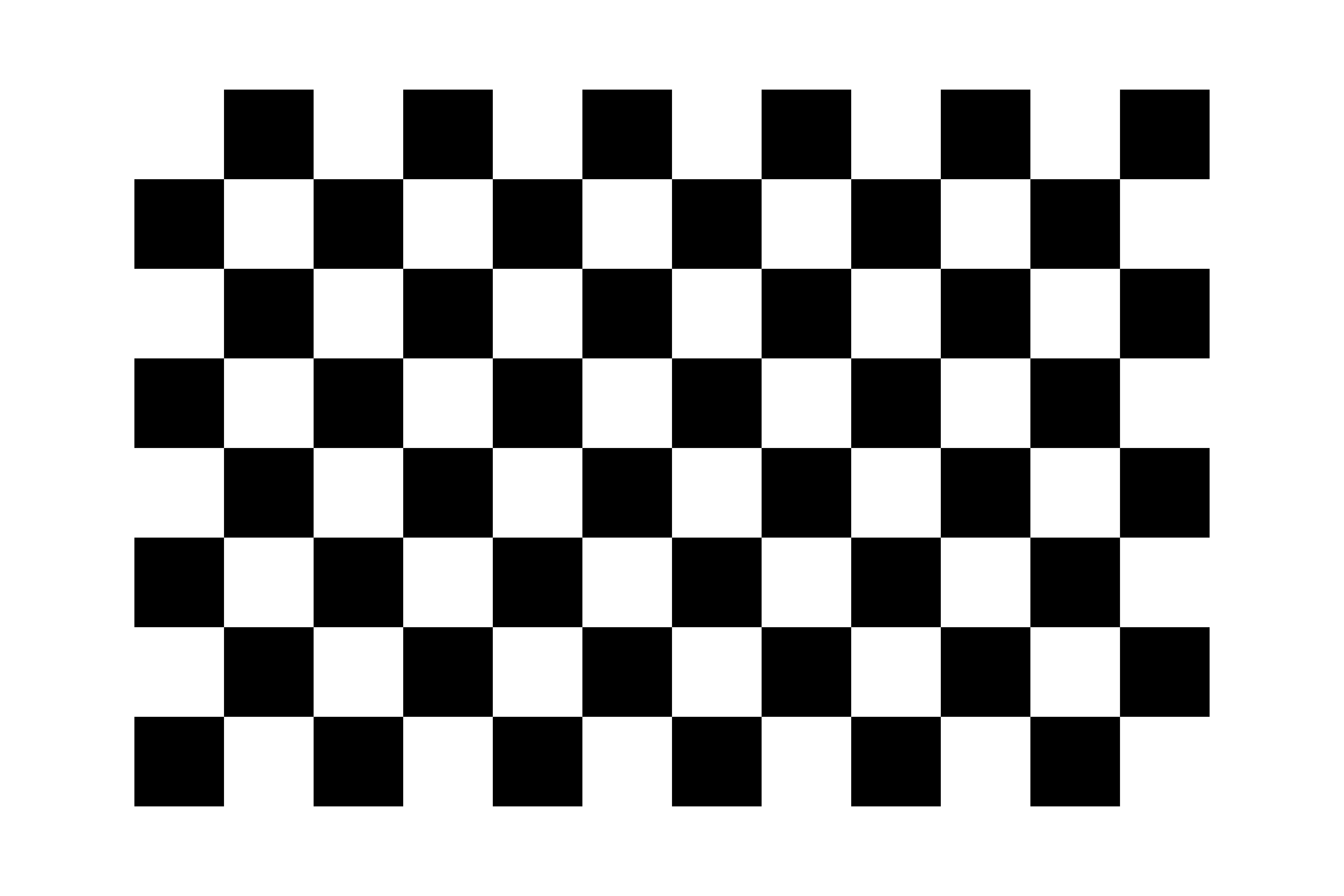# 图像标定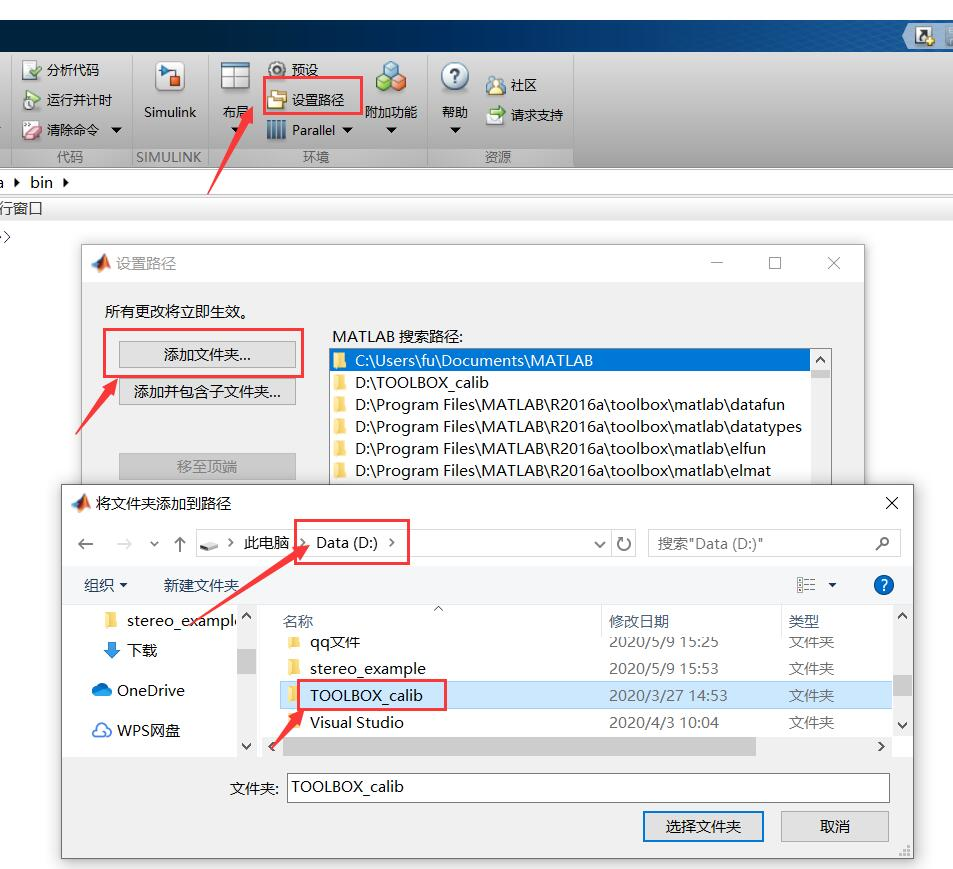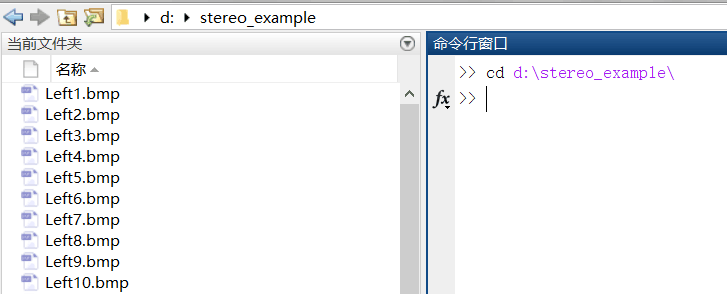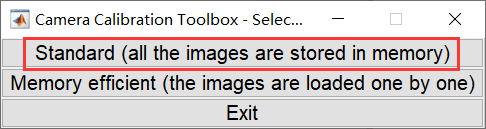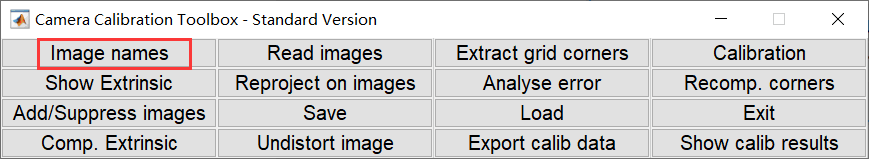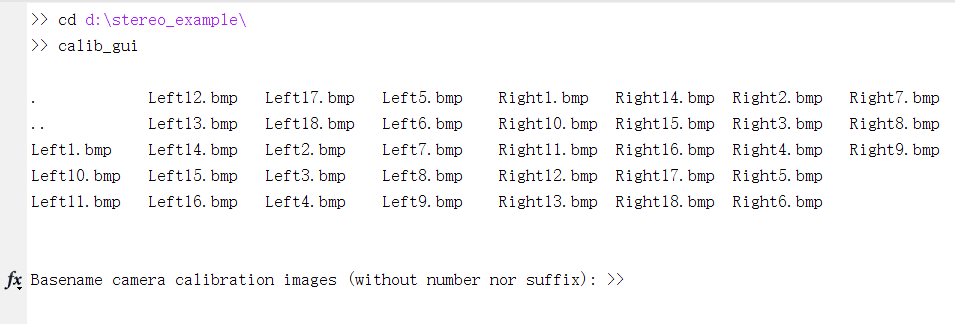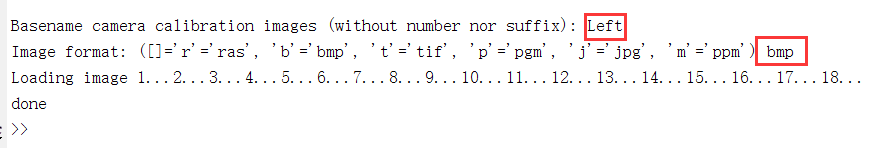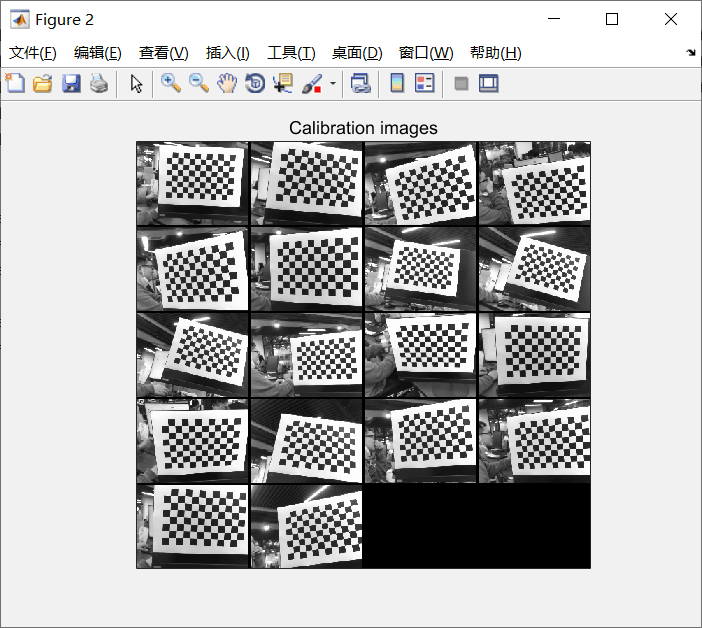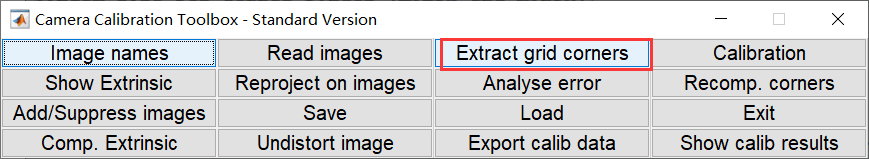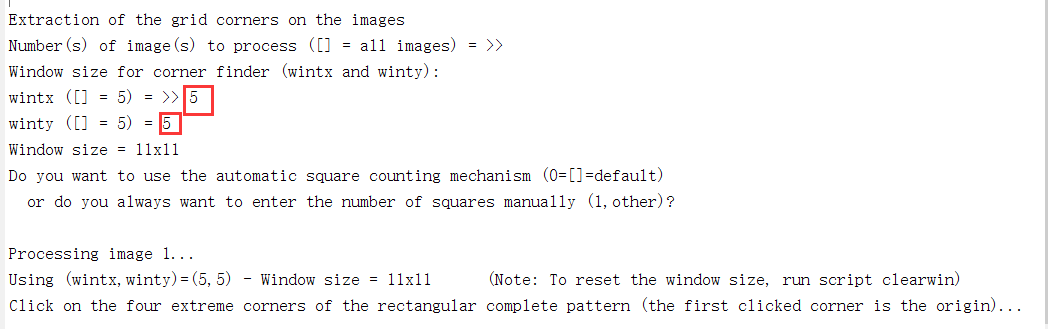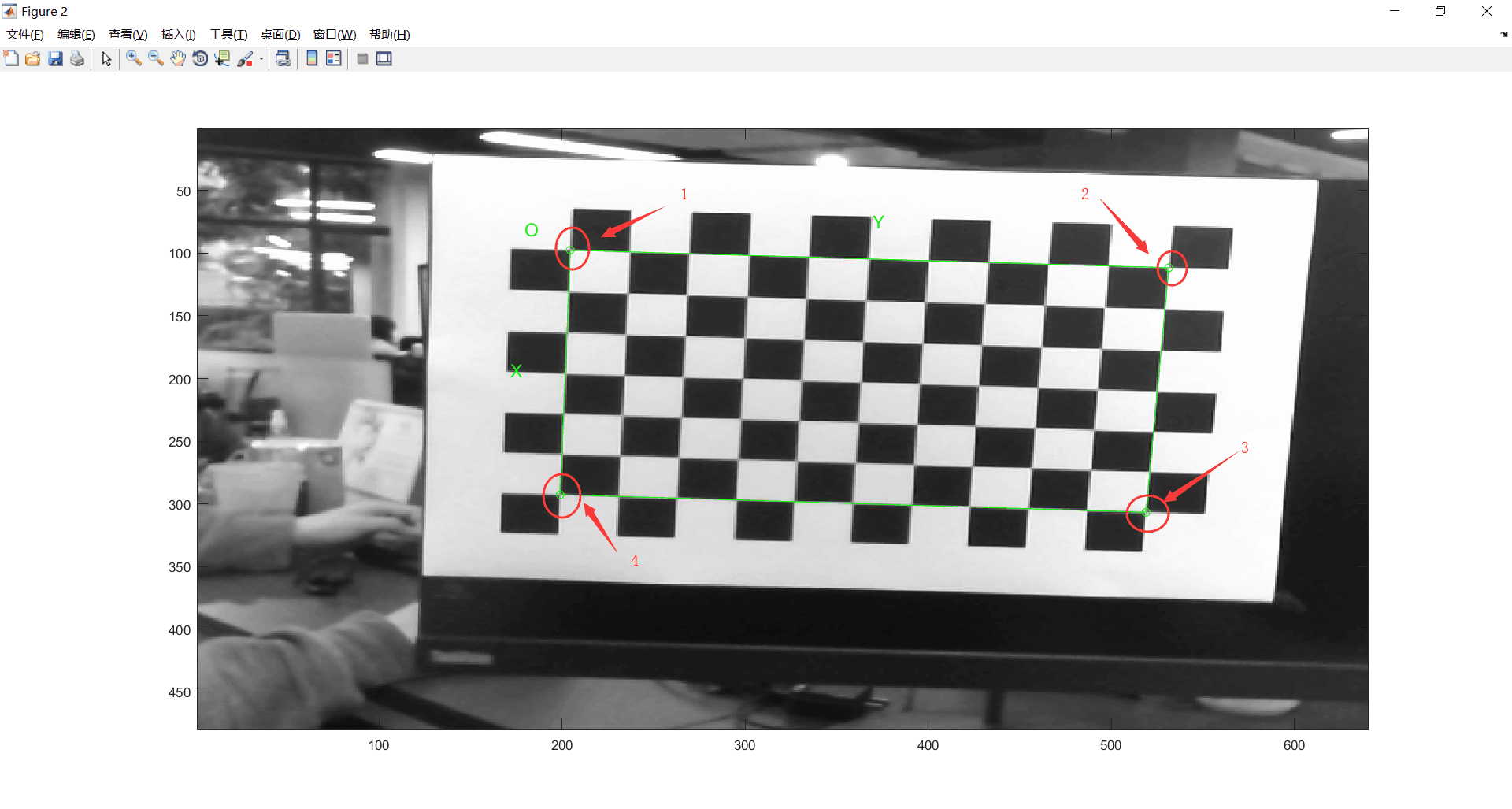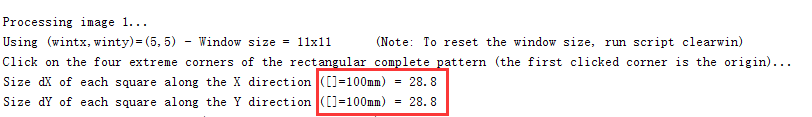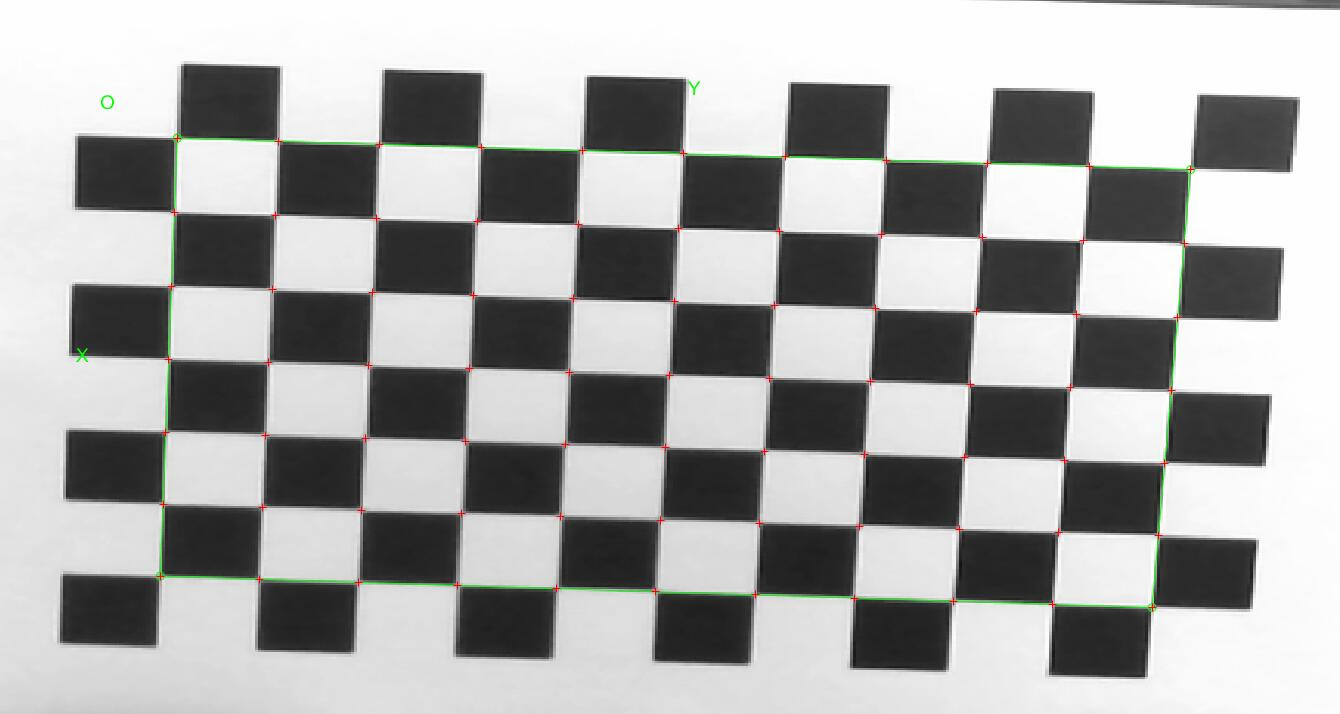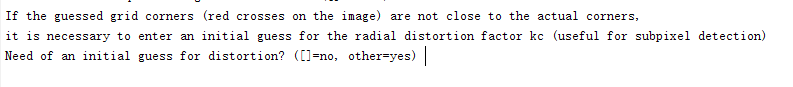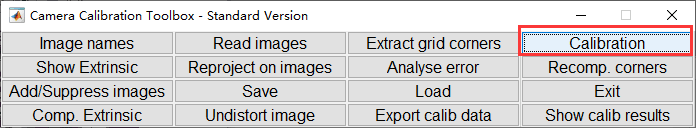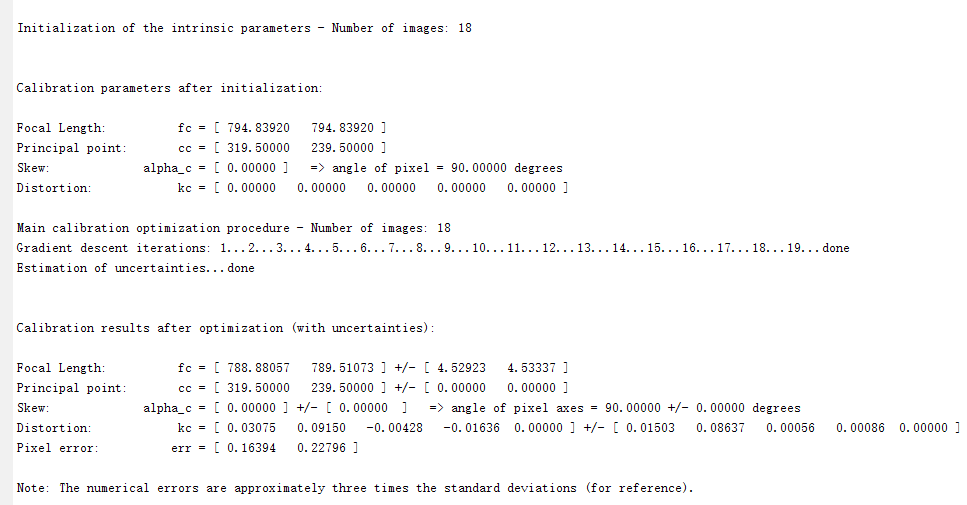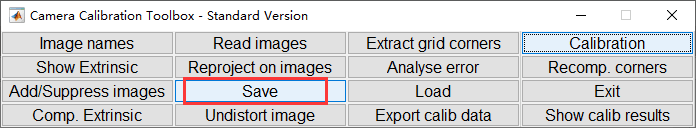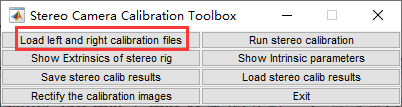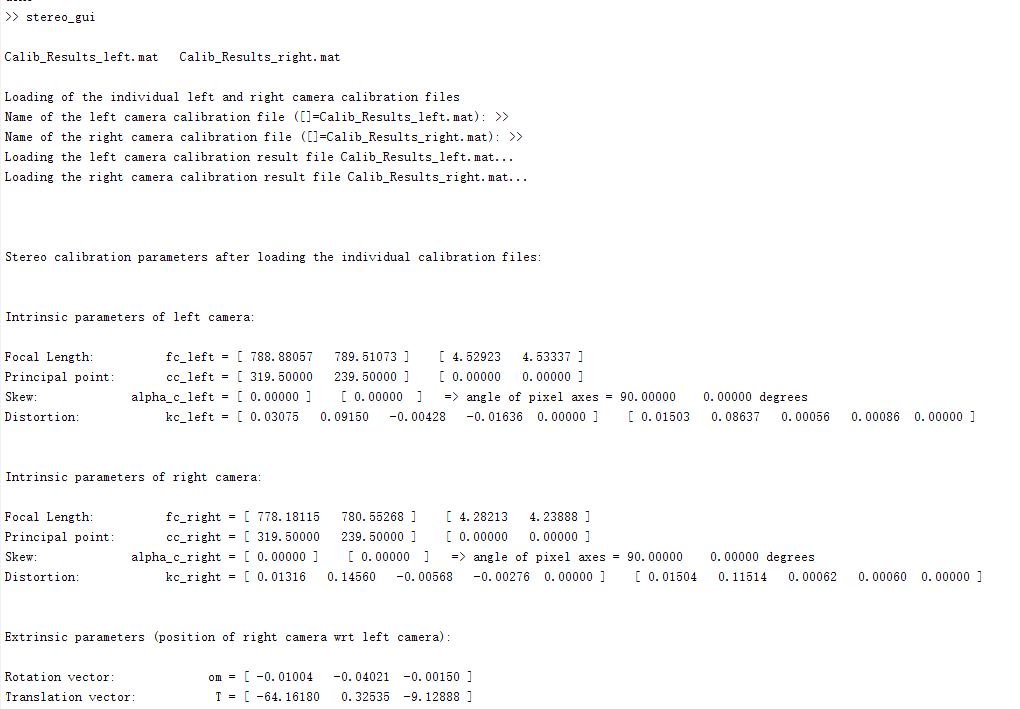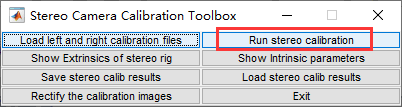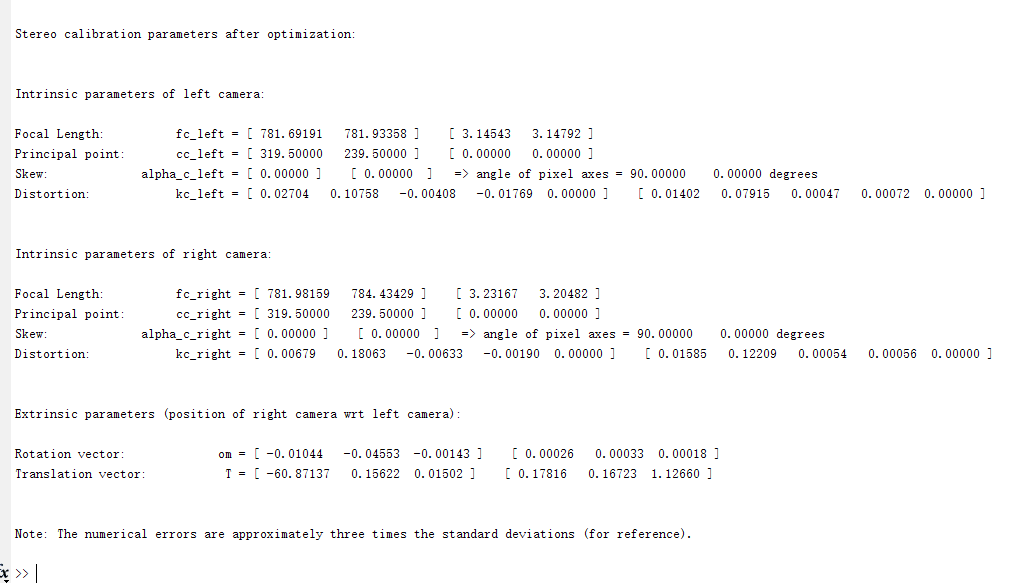fc_left：左目镜头像素级焦距值，fc_left × 像元尺寸 = 左目镜头物理焦距值，像

cc_left：左目光心位置坐标。
kc_left：左目镜头畸变系数。
alpha_c_left: 偏斜系数。
om：旋转向量。
T：平移向量。T_01，双目间距（即：双目基线）

Focal Length:          fc_left = [ 781.69191   781.93358 ]  [ 3.14543   3.14792 ]
【fc_left_x     fc_left_y】      【误差1，  误差2】
Principal point:       cc_left = [ 319.50000   239.50000 ]   [ 0.00000   0.00000 ]
【cc_left_x     cc_left_y】     【误差1     误差2】
Skew:             alpha_c_left = [ 0.00000 ]   [ 0.00000  ]   => angle of pixel axes = 90.00000   0.00000 degrees
Distortion:            kc_left = [ 0.02704   0.10758   -0.00408   -0.01769  0.00000 ]   [ 0.01402   0.07915   0.00047   0.00072  0.00000 ]
【kc_left_01,  kc_left_02,  kc_left_03,  kc_left_04,   kc_left_05】【误差1 误差2 误差3 误差4 误差5】

Intrinsic parameters of right camera:

Focal Length:          fc_right = [ 781.98159   784.43429 ]   [ 3.23167   3.20482 ]
【fc_right_x     fc_right_y】      【误差1，  误差2】
Principal point:       cc_right = [ 319.50000   239.50000 ]   [ 0.00000   0.00000 ]
【cc_right_x     cc_right_y】     【误差1     误差2】
Skew:             alpha_c_right = [ 0.00000 ]   [ 0.00000  ]   => angle of pixel axes = 90.00000   0.00000 degrees
Distortion:            kc_right = [ 0.00679   0.18063   -0.00633   -0.00190  0.00000 ]   [ 0.01585   0.12209   0.00054   0.00056  0.00000 ]
【kc_right_01,  kc_right_02,  kc_right_03,  kc_right_04,   kc_right_05】【误差1 误差2 误差3 误差4 误差5】

Extrinsic parameters (position of right camera wrt left camera):

【rec旋转向量】
Rotation vector:             om = [ -0.01044   -0.04553  -0.00143 ]   [ 0.00026   0.00033  0.00018 ]

【T平移向量】
Translation vector:           T = [ -60.87137   0.15622  0.01502 ]   [ 0.17816   0.16723  1.12660 ]


# 距离测量

SGBM算法在OpenCV中已经开源，该算法的函数为cv2.StereoSGBM_create。下面我们就使用Python实现这个双目测距的程序，为了简单，该程序只是使用本地保存的左目图像和右目图像，如何读者想使用摄像头拍摄，可以参考文章开头提供的拍照代码，两者结合，实时检测距离。

import cv2
import numpy as np

imageWidth = 1280
imageHeight = 720
imageSize = (imageWidth, imageHeight)


'''左目相机标定参数
fc_left_x   0            cc_left_x
0           fc_left_y    cc_left_y
0           0            1
'''
cameraMatrixL = np.array([[849.38718, 0, 720.28472],
[0, 850.60613, 373.88887],
[0, 0, 1]])
# [kc_left_01,  kc_left_02,  kc_left_03,  kc_left_04,   kc_left_05]
distCoeffL = np.array([0.01053, 0.02881, 0.00144, 0.00192, 0.00000])

'''右目相机标定参数
fc_right_x   0              cc_right_x
0            fc_right_y     cc_right_y
0            0              1
'''
cameraMatrixR = np.array([[847.54814, 0, 664.36648],
[0, 847.75828, 368.46946],
[0, 0, 1]])

# kc_right_01,  kc_right_02,  kc_right_03,  kc_right_04,   kc_right_05
distCoeffR = np.array([0.00905, 0.02094, 0.00082, 0.00183, 0.00000])

# T平移向量
T = np.array([-59.32102, 0.27563, -0.79807])

# rec旋转向量
rec = np.array([-0.00927, -0.00228, -0.00070])


# 立体校正
R = cv2.Rodrigues(rec)
Rl, Rr, Pl, Pr, Q, validROIL, validROIR = cv2.stereoRectify(cameraMatrixL, distCoeffL, cameraMatrixR, distCoeffR,
imageSize, R, T, flags=cv2.CALIB_ZERO_DISPARITY, alpha=0,
newImageSize=imageSize)

# 计算更正map
mapLx, mapLy = cv2.initUndistortRectifyMap(cameraMatrixL, distCoeffL, Rl, Pl, imageSize, cv2.CV_32FC1)
mapRx, mapRy = cv2.initUndistortRectifyMap(cameraMatrixR, distCoeffR, Rr, Pr, imageSize, cv2.CV_32FC1)

# 读取图片
grayImageL = cv2.cvtColor(rgbImageL, cv2.COLOR_BGR2GRAY)
grayImageR = cv2.cvtColor(rgbImageR, cv2.COLOR_BGR2GRAY)

# 经过remap之后，左右相机的图像已经共面并且行对齐
rectifyImageL = cv2.remap(grayImageL, mapLx, mapLy, cv2.INTER_LINEAR)
rectifyImageR = cv2.remap(grayImageR, mapRx, mapRy, cv2.INTER_LINEAR)

# SGBM算法重要的参数
mindisparity = 32
ndisparities = 176
# 惩罚系数

# BM算法
sgbm.setP1(P1)
sgbm.setP2(P2)

sgbm.setPreFilterCap(60)
sgbm.setUniquenessRatio(30)
sgbm.setSpeckleRange(2)
sgbm.setSpeckleWindowSize(200)
sgbm.setDisp12MaxDiff(1)
disp = sgbm.compute(rectifyImageL, rectifyImageR)

# 在实际求距离时，ReprojectTo3D出来的X / W, Y / W, Z / W都要乘以16
xyz = cv2.reprojectImageTo3D(disp, Q, handleMissingValues=True)
xyz = xyz * 16


xyz是一个矩阵，不能直观体现图像中物体的距离，所以我们可以把他们转换成一张灰度图，更加颜色的深浅就知道他们的大概情况了。然后我们还可以添加一个鼠标点击事件，这样通过点击图像上的点，直接输出该点的三维坐标。

# 用于显示处理
disp = disp.astype(np.float32) / 16.0
disp8U = cv2.normalize(disp, disp, alpha=0, beta=255, norm_type=cv2.NORM_MINMAX, dtype=cv2.CV_8U)
disp8U = cv2.medianBlur(disp8U, 9)

# 鼠标点击事件
def onMouse(event, x, y, flags, param):
if event == cv2.EVENT_LBUTTONDOWN:
print('点 (%d, %d) 的三维坐标 (%f, %f, %f)' % (x, y, xyz[y, x, 0], xyz[y, x, 1], xyz[y, x, 2]))

# 显示图片
cv2.imshow("disparity", disp8U)
cv2.setMouseCallback("disparity", onMouse, 0)

cv2.waitKey(0)
cv2.destroyAllWindows()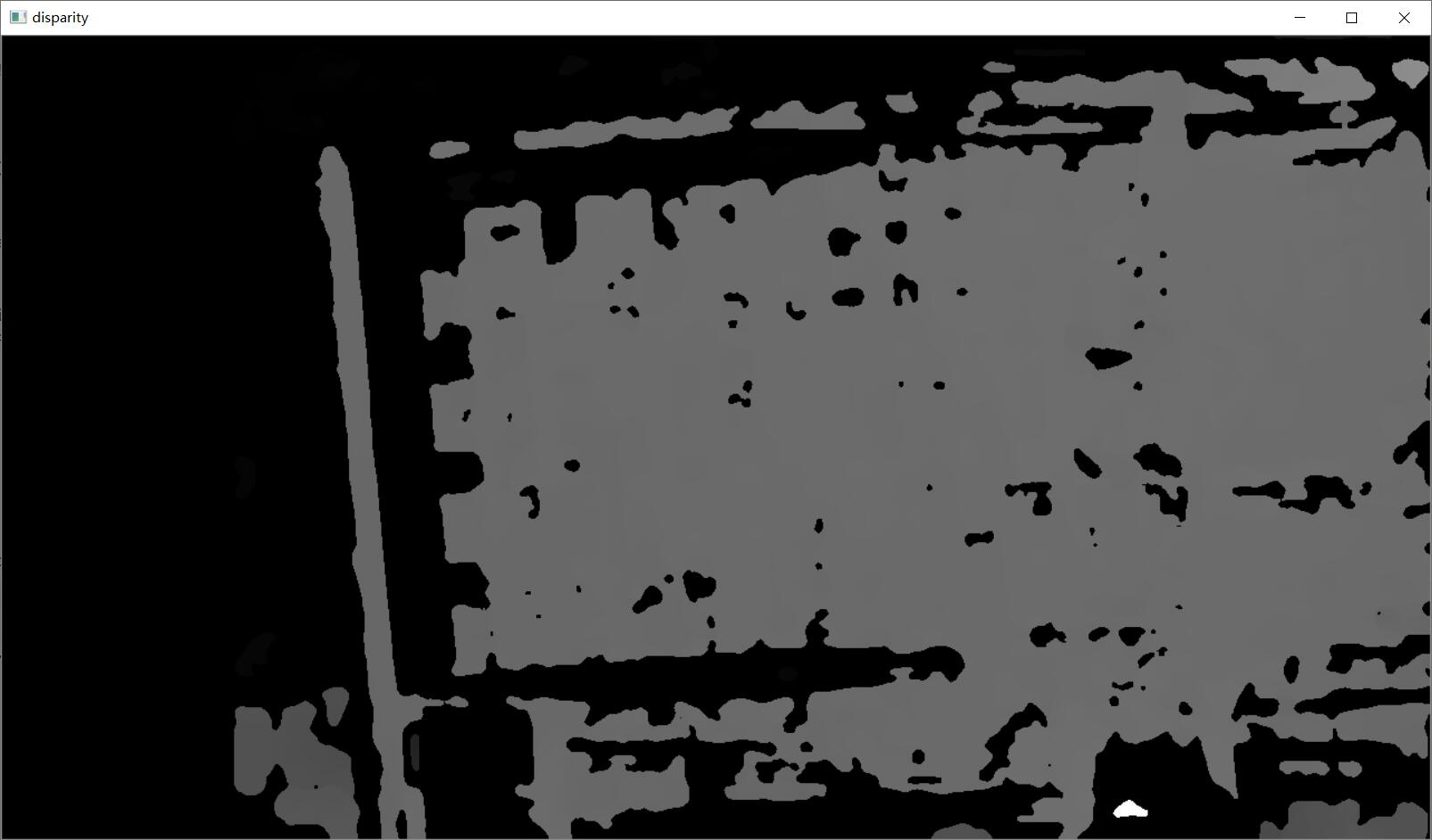点 (777, 331) 的三维坐标 (57.852905, -23.240721, 519.846985)



07-151927
05-231万+08-102147
08-147553
10-208690
03-11578
07-14
05-066万+